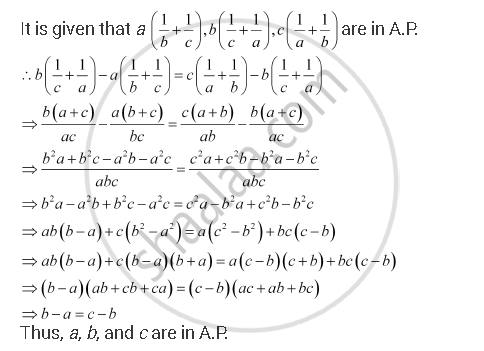CBSE (Arts) Class 11CBSE
Share

# If A(1/B + 1/C), B(1/C+1/A), C(1/A+1/B) Are in A.P., Prove That A, B, C Are in Arithmetic Progression - CBSE (Arts) Class 11 - Mathematics

ConceptArithmetic Progression (A.P.)

#### Question

if a(1/b + 1/c), b(1/c+1/a), c(1/a+1/b) are in A.P., prove that a, b, c are in A.P.

#### SolutionIs there an error in this question or solution?

#### APPEARS IN

NCERT Solution for Mathematics Textbook for Class 11 (2018 to Current)
Chapter 9: Sequences and Series
Q: 16 | Page no. 199

#### Video TutorialsVIEW ALL 

Solution If A(1/B + 1/C), B(1/C+1/A), C(1/A+1/B) Are in A.P., Prove That A, B, C Are in Arithmetic Progression Concept: Arithmetic Progression (A.P.).
S